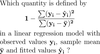Level 25 Level 27
Level 26

## Ignore words

Check the boxes below to ignore/unignore words, then click save at the bottom. Ignored words will never appear in any learning session.

Ignore?
Linear regression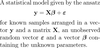Dependent variable / regressand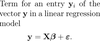Independent variable / regressor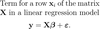Design matrix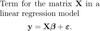Regression coefficient / effect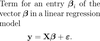Error term / noise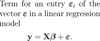Intercept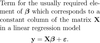Polynomial regression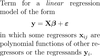Total sum of squares / TSS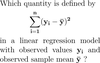Explained sum of squares / ESS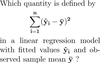Residual sum of squares / RSS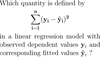Intercept (has to exist)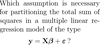Hat matrix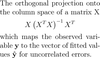Coefficient of determination / R^2